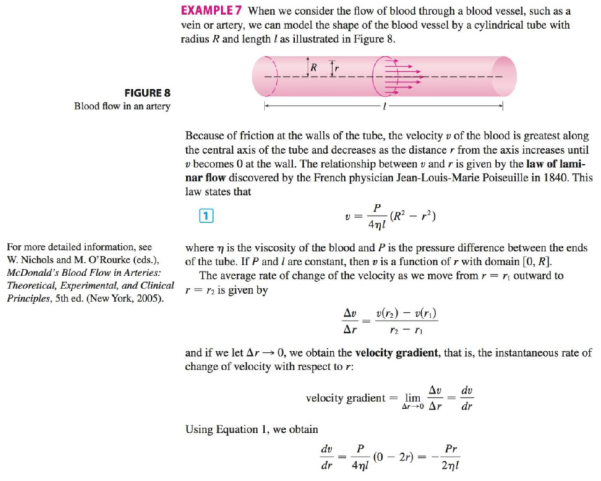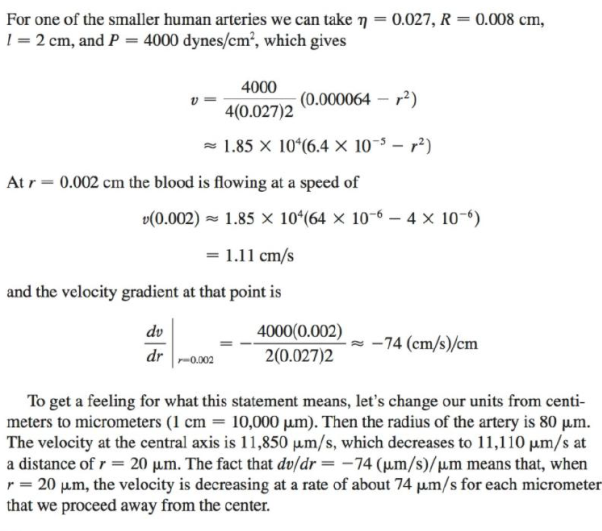Chapter 3.7, Problem 29E

Chapter
Section
Textbook Problem

Refer to the law of laminar flow given in Example 7. Consider a blood vessel with radius 0.01 cm, length 3 cm, pressure difference 3000 dynes/cm2, and viscosity η = 0.027.(a) Find the velocity of the blood along the center-line r = 0, at radius r = 0.005 cm, and at the wall r = R = 0.01 cm.(b) Find the velocity gradient at r = 0, r = 0.005, and r = 0.01.(c) Where is the velocity the greatest? Where is the velocity changing most?EXAMPLE 7(a)

To determine

To find: The velocity of the blood along the center line r=0, at radius r=0.005cm and at the wall r=R=0.01cm.

Explanation

Given:

The law of laminar flow is as given below.

v(r)=P4ηl(R2r2) (1)

The length of the blood vessel is given below.

l=3cm

The pressure difference between the ends of the vessel is given below.

P=3000dynes/cm2

The viscosity of the blood is given below.

η=0.027

Calculation:

Calculate the velocity of the blood along the center line using the equation (1).

v(r)=P4ηl(R2r2)

Substitute 3cm for l, 0 for r, 3000dynes/cm2 for P, 0.027 for η and 0.01cm for R in the equation (1).

v(0)=30004×0.027×3(0.0120)=9259.25×0.0001

v(0)=0.925cm/s

Thus, the Velocity of the blood at r=0cm is 0.925cm/s.

Calculate the velocity of the blood at r=0.005cm using the equation (1).

v(r)=P4ηl(R2r2)

Substitute 3 cm for l, 0

(b)

To determine

To find: The velocity gradient at r=0, r=0.005 and r=0.01.

(c)

To determine

To find: Where is the velocity the greatest and where it changes the most.

Still sussing out bartleby?

Check out a sample textbook solution.

See a sample solution

The Solution to Your Study Problems

Bartleby provides explanations to thousands of textbook problems written by our experts, many with advanced degrees!

Get Started

Explain in your own words the meaning of limxf(x)=L and limxf(x)=M.

Applied Calculus for the Managerial, Life, and Social Sciences: A Brief Approach

The arc length function for y = x2, 1 x 3 is s(x) = a) 1x1+4x2dx b) 1x1+4t2dt c) 1x1+t4dt d) 1x1+4t4dt

Study Guide for Stewart's Single Variable Calculus: Early Transcendentals, 8th

0 1 π It does not exist.

Study Guide for Stewart's Multivariable Calculus, 8th